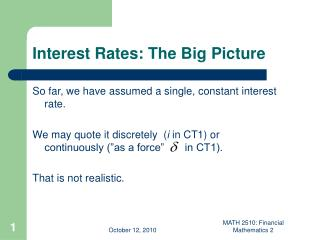DownloadDownload PresentationInterest Rates: The Big Picture

# Interest Rates: The Big Picture

Download Presentation## Interest Rates: The Big Picture

- - - - - - - - - - - - - - - - - - - - - - - - - - - E N D - - - - - - - - - - - - - - - - - - - - - - - - - - -
##### Presentation Transcript

1. Interest Rates: The Big Picture So far, we have assumed a single, constant interest rate. We may quote it discretely (i in CT1) or continuously (”as a force” in CT1). That is not realistic. MATH 2510: Financial Mathematics 2

2. Danish Interest Rates MATH 2510: Financial Mathematics 2

3. Interest rates are ”non-constant in two dimensions”: Term (or time to maturity) and calendar time. In CT1, Units 13 and 14 we relax the assumption MATH 2510: Financial Mathematics 2

4. The Term Structure of Interest Rates CT1, Unit 13 Sec. 1-3: Definitions and concepts • (n-year) zero coupon bonds and (discrete) spot rates • Discrete forward rates; intepretation and realtion to spot rates • Continuous versions of the rates • Instantaneous forward rates MATH 2510: Financial Mathematics 2

5. Zero coupon bonds An n-term zero coupon bond (ZCB) pays £1 after n years – and nothing else. Its price is denoted by Pn. ZCBs differ by term. If we know ZCB prices, we can easily price anything else w/ deterministic payments: MATH 2510: Financial Mathematics 2

6. Spot Rates/Zero Coupon Rates The discrete spot rate, yn, is the yield on an n-term zero coupon bond: The function that maps term n to spot rate ynis called the (zero coupon) yield curve or the term structure of interest rates MATH 2510: Financial Mathematics 2

7. Forward Rates The discrete forward rate ft,r is the interest rate that can be agreed upon today for a future loan that runs between time t and time t+r. At time t, we recieve £1, at time t+r we pay back £1*(1+ ft,r)r. (And no money changes hands today.) MATH 2510: Financial Mathematics 2

8. An (absence of) arbitrage argument shows that forward rates and zero coupon bond prices are related We write ft for the one-period forward rates ft,1 ,by repeatedly using the formula above we see that Spot rates are (geometric’ish) averages of forward rates. MATH 2510: Financial Mathematics 2

9. The Yield Curve CT1, Unit 13, Sec. 4.1 Real-world yield curves come in many shapes • Increasing w/ term (”normal”; Fig. 2, Unit 13) • Decreasing w/ term (”inverted”; Fig. 1) • Humped (Fig. 3) MATH 2510: Financial Mathematics 2

10. Five Danish Yield Curves MATH 2510: Financial Mathematics 2

11. Why do Yield Curves Look the Way the Do? Popular explanations (not ”models”) • Expectations Theory. Rates will fall. I’d better lock in a good rate on my long term investments. Higher demand for long term bonds -> higher prices -> lower yields. Good for inverted yield curves. MATH 2510: Financial Mathematics 2

12. Liquidity Preference: Higher long term rates is a compensation for risk. (Or: Lower short term rates is a reward for short term funding.) Does actually have ”theoretical merit”. • Market Segmetation. Supply and demand are important. But also ”Humped curve? I give up!” MATH 2510: Financial Mathematics 2